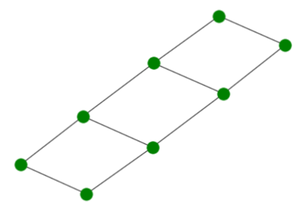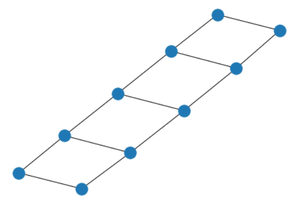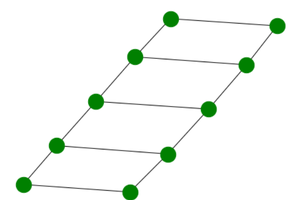Open In App

# Ladder Graph Using Networkx Module in Python

In this article, we are going to see the ladder graph using Python. It is a graph that looks like ladders used commonly with every node attached to two other nodes in a specific manner. We can obtain a ladder graph by joining two-path graphs of n nodes each by each node connected with a corresponding node in another path graph.

Representation:

Below attached is an image of the L4 (n) Ladder Graph that Returns the Ladder graph of length 4(n).• It is an undirected graph.
• It is Planar
• An Ln ladder graph has 2n. nodes.
• Chromatic number of a ladder graph is 2.
• An Ln ladder graph has 3n-2 edges.
• It is a Hamiltonian graph
• It is a Connected graph.
• The ladder graph is a Bipartite graph.

We will use the networkx module for realizing a Ladder graph. It comes with an inbuilt function networkx.ladder_graph() and can be illustrated using the networkx.draw() method.

Syntax: networkx.draw(G, node_size, node_color)

Parameters:

• G: It refers to the ladder graph object
• node_size: It refers to the size of nodes.
• node_color: It refers to color of the nodes.

Below are some examples to depict how to illustrate a Ladder graph in Python:

Approach:

• We will import the required networkx module.
• After that, we will initialize a number of nodes to 5.
• We will create graph object G using ladder_graph() function.
• We will realize the graph using nx.draw() function.

Example 1:

## Python3

 `# import required module``import` `networkx` `# number of nodes``n ``=` `5` `# create object``G ``=` `networkx.ladder_graph(n)` `# illustrate graph``networkx.draw(G)`

Output:Example 2: Making color of nodes green and increasing size by passing extra arguments to nx.draw() function as discussed above.

Approach:

• We will import the required networkx module.
• After that, we will initialize number of nodes to 5.
• We will create graph object G using ladder_graph() function.
• We will realize the graph using nx.draw() function.
• We will make the color of nodes green and increasing size by passing extra arguments to nx.draw()

## Python3

 `# import required module``import` `networkx` `# create object``G ``=` `networkx.ladder_graph(``5``)` `# illustrate graph``networkx.draw(G, node_size ``=` `500``,``            ``node_color ``=` `'green'``)`

Output: